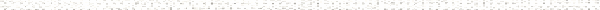A Precalculus Outline

 Chapter 2:  Linear and Quadratic FunctionsObjective: Finding the slope between two pointsObjective: Finding the equation of a line given information about the lineObjective: Writing a line in slope-intercept form to graphObjective: Graphing a line given in slope-intercept formObjective: Graphing horizontal linesObjective: Graphing vertical linesObjective: Interpreting slope as a rate of changeObjective: Writing Linear ModelsObjective: Finding the Average Rate of Change of a FunctionObjective: Plotting points in the plane.Objective: X-interceptsObjective: Y-interceptsObjective: Solving linear equations Section 2.2:  Absolute Value FunctionsObjective: Definition of absolute valueObjective: Piecewise definition of absolute valueObjective: Writing a function involving absolute values as a piecewise-defined functionObjective: Solving equations involving absolute valueObjective: Graphing absolute value functionsObjective: Graphing piecewise defined functionsObjective: X-interceptsObjective: Y-interceptsObjective: Finding domain of a function graphicallyObjective: Finding range of a function graphicallyObjective: Finding a relative maximum of a function graphicallyObjective: Finding a relative minimum of a function graphicallyObjective: Finding a local maximum of a function graphicallyObjective: Finding a local minimum of a function graphicallyObjective: Finding the maximum of a function graphicallyObjective: Graphing Quadratic Functions by plotting pointsObjective: Graphing a quadratic function by converting it to standard form by completing the squareObjective: Graphing a quadratic function by finding its zeros and using the vertex formulaObjective: Using the vertex formula to find the vertex of the graph of a quadratic functionObjective: Solving a quadratic equation using the quadratic formulaObjective: Finding the discriminant of a quadratic equation and using it to characterize the nature of solutionsObjective: Solving application problems featuring quadratic functionsObjective: Solving a quadratic equation by completing the squareObjective: X-interceptsObjective: Y-interceptsObjective: Finding domain of a function graphicallyObjective: Finding range of a function graphicallyObjective: Finding a relative maximum of a function graphicallyObjective: Finding a relative minimum of a function graphicallyObjective: Finding a local maximum of a function graphicallyObjective: Finding a local minimum of a function graphicallyObjective: Finding the maximum of a function graphically Section 2.4:  Inequalities with Absolute Value and Quadratic FunctionsObjective: Solving inequalities graphicallyObjective: Solving inequalities involving absolute valueObjective: Solving quadratic inequalities using a sign diagramObjective: Graphing inequalities in two variablesObjective: Using inequalities to describe regions in the planeObjective: Graphing functionsObjective: Solving linear inequalitiesObjective: Solving compound inequalitiesObjective: Intersection of setsObjective: Union of setsObjective: Using a calculator to find the least squares regression lineObjective: Using a calculator to create a quadratic regression modelObjective: Plotting points in the planeObjective: Slope as a rate of changeObjective: Vertex of a quadratic functionObjective: Vertex of a parabola